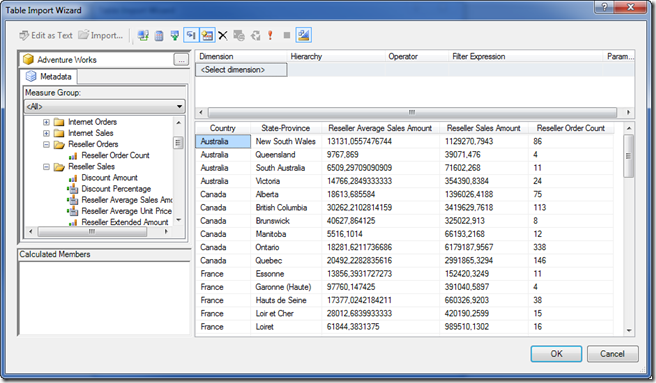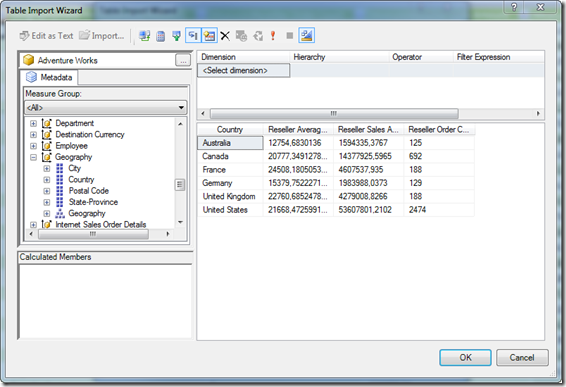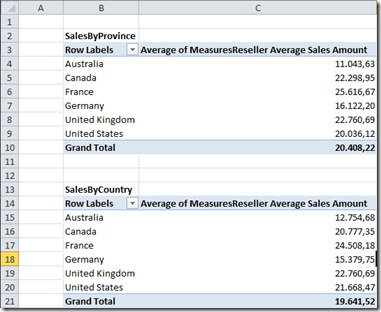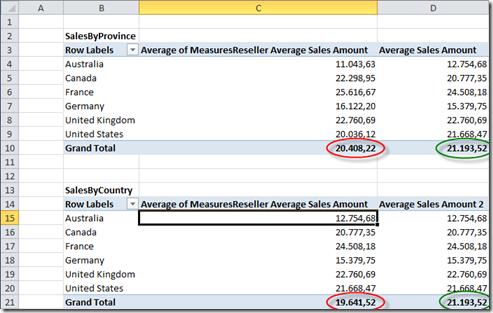A general problem of any cube is the way PowerPivot handles calculated members. From a technical point of view, it is absolutely correct that PowerPivot imports the result of a calculated member evaluated at the granularity of the query result. However, from the user point of view, it is not pretty clear why importing a calculated member it imports a value and not the formula. If your users are used to browse cubes, and because calculated member are defined at the cube level and not on the client side, users often don’t see the difference between a “native” measure and a calculated one. For example, if a calculated member shows the value of an indicator (for example, the ratio of sales per phone call), when a user imports that indicator in PowerPivot for Country, City, Year, Month, Day and Product, he will see a wrong number looking at this indicator browsing data and selecting only the total per Year, without a Month/Day detail.

The user would expect to import also the logic of a calculated member into PowerPivot and not just the resulting value, even if from a technical point of view the actual behavior is correct. Moreover, the fact that end users see correct data in the PowerPivot table might induce him to not check how that column calculation will behave while browsing a PivotTable.

We can reproduce the typical scenario also by using AdventureWorks cube. If you look at Figure 1 you can see data selected for import:

• Geography.Country.Country
• Geography.[State-Province].[State-Province]
• Reseller Average Sales Amount (calculated member)
• Reseller Sales Amount
• Reseller Order CountFigure 1 – Query Designer importing data by Country and State-Province

The Reseller Sales Amount and Reseller Order Count measures are used to calculate the Reseller Average Sales Amount measure. We know that into the cube there is a definition like:

[Reseller Average Sales Amount] = SUM( [Reseller Sales Amount] ) / SUM( [Reseller Order Count] )

However, the end user cannot see this definition in any place of the wizard and might be difficult for him to find the [Reseller Order Count] measure, which is into a different folder (Reseller Orders instead of Reseller Sales). Moreover, in this case both terms are visible measures, but often (and it happens also to the customer) one or more measures used to make a calculation are hidden to the end users, making it almost impossible to find the measure to use in the calculation. You can see in Figure 2 that another user imported data from the same data source, but using only the Country and not the Province-State attribute.Figure 2 – Query Designer importing data by Country only

When the user compares the two tables aggregated by Country in PivotTable, he sees different values, like you can see in Figure 3. Despite the tentative to use Average to display a meaningful value for the Grand Total, all the values for all the Countries are different.Figure 3 – PivotTable of the two tables imported in PowerPivot

If you make the calculation by dividing the Reseller Sales Amount by the Reseller Order Count, you can see in the column D of the worksheets shown in Figure 4 that the number is calculated correctly for Grand Total in both cases. Because the extraction was made at the Country level, also the values for each Country are right in the SalesByCountry pivot table, but values are different in SalesByProvince PivotTable because each Country shows the average of the value returned from the cube for each province.Figure 4 – Differences between averages of PowerPivot measure (column C) and calculated measure (column D)

Thus, only the definition of a measure with a DAX expression can display right information. This is a very simple calculation and is a widely spread issue in the cubes of the customer.

### Final consideration

I hope to see improvements in this area in future versions of PowerPivot. By now, it is necessary to instruct users about how to build the calculation in PowerPivot, providing them the necessary raw measure that allow building a DAX expression that produces the same result.